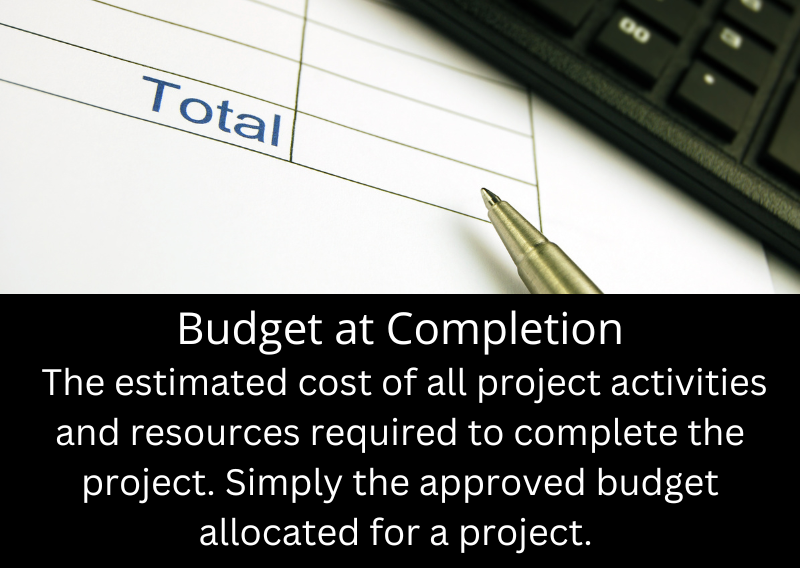# What does Budget at Completion mean?

### Budget at Completion

Budget at Completion (BAC) is a term used in project management to represent the total approved budget allocated for a project. It is the estimated cost of all project activities and resources required to complete the project successfully.
BAC is a baseline for measuring project performance, specifically in the context of Earned Value Management (EVM).## What is the formula for Budget at Completion?

Formula:
BAC = Σ (Planned Costs for Each Activity)

Where Σ represents the total planned costs for each activity or work package in the project.

Example Calculation:
Let's assume a project with four activities, with the following planned costs for each activity:

Activity A: \$10,000
Activity B: \$15,000
Activity C: \$20,000
Activity D: \$5,000
To calculate the Budget at Completion (BAC) for this project, we simply add up the planned costs of each activity:

BAC = Activity A + Activity B + Activity C + Activity D
BAC = \$10,000 + \$15,000 + \$20,000 + \$5,000
BAC = \$50,000

In this example, the project's Budget at Completion (BAC) is \$50,000.

## What estimating methods can you use to calculate the BAC?

There are several ways to estimate the cost of each activity or work package, before adding them up to get the BAC.

1. Analogous Estimating: This method uses historical data from similar past projects to estimate the cost of the current project. It is a top-down approach useful when limited project-specific information is available. However, the accuracy of this method depends on the similarity between the past and current projects.

2. Parametric Estimating: This method uses statistical relationships between historical data and other variables (e.g., square footage, hours, or complexity) to calculate cost estimates. It is useful when reliable, project-specific data is available and can provide accurate estimates if the underlying relationships and data are sound.

3. Bottom-Up Estimating: This method involves estimating the cost of individual work packages or activities and then aggregating these costs to obtain the BAC. This approach is more accurate compared to top-down methods, as it considers detailed project information. However, it can be time-consuming, especially for large projects.

4. Three-Point Estimating: This method uses three estimates for each activity – optimistic, most likely, and pessimistic – to account for uncertainties and risks. The three estimates are combined using either the triangular or beta (PERT) distribution formula to calculate each activity's average cost estimate. Finally, adding up these estimates produces the BAC.

5. Expert Judgment: This method involves consulting experts or experienced team members to provide cost estimates for project activities. Expert judgment can be combined with other estimating techniques to increase the accuracy of cost estimates.

Once you have estimated the cost of each activity or work package using one or more of these methods, you can add them up to calculate the project's Budget at Completion (BAC).

See all project management words